GRE Subject Test: Chemistry : Gravimetric Analysis

Example Questions

Example Question #41 : Analytical Chemistry

Household vinegar contains the organic compound acetic acid with chemical formula,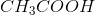. If a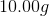vinegar sample contains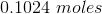of acetic acid, calculate the percent (mass/mass) of thein the vinegar sample.

Density of vinegar is the following: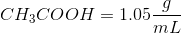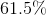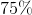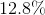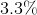Explanation:

Convert the moles ofto grams: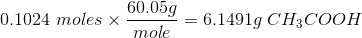To calculate the percentage ofin the vinegar we need to use the following formula: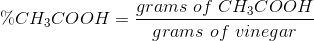Therefore,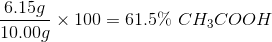All GRE Subject Test: Chemistry Resources